# General RLC circuit - Current through inductor

#### gianx80

Joined Jan 23, 2011
14
Hi to all and thanks in advance. I'm studying for one of my exams (I work so I can't attend lessons and the Professor doesn't reply to my emails).
In the following circuit I have to find the current through the inductor: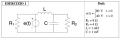for t < 0 we have: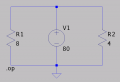So IR1 = 10A, IR2 = 20A. Current through the inductor is 20A and the voltage across capacitor is 80V.

for t = ∞ we have: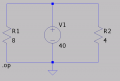So IR1 = 5A, IR2 = 10A. Current through the inductor is 10A and the voltage across capacitor is 40V.

for t = 0+ we have the initial circuit, the current though inductor is 20A and the voltage across the capacitor is 80V.
So we have a soluzione like this one: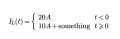now, the circuit is neither RLC parallel or series, right? Can I lead it back to a know simpler case? Am I doing something wrong?

I found examples with combination of series and parallels, but they use phasors and, in this case, I don't have a AC source (so I cannot compute impedance).

I found examples of RC or RL circuits solved used Thevenin, again, if I use the inductor as a load I have the parallel of a capacitor and a resistor, so no way.

How can I proceed?

Thanks, I'm desperate

#### MrAl

Joined Jun 17, 2014
10,586
Do you only need solution for t<0 and t-->inf or entire time response?

#### WBahn

Joined Mar 31, 2012
29,137
Hopefully you recognize that one of the components in the circuit can be removed completely as it has absolutely no effect on the current in the inductor at any time. Do you see which component and why?

Have you started learning about Laplace transforms yet? You don't need them so solve this problem, but it makes it a lot easier.

#### gianx80

Joined Jan 23, 2011
14
Do you only need solution for t<0 and t-->inf or entire time response?
I don't know, I don't have the solutions. It asks for: "Find the current through the inductor in every moment. Professor doesn't reply to me, so I don't know more than that. I used a solution like this because I found a simpler exercise on the Internet that calculated for t<0 and t >= 0.

Hopefully you recognize that one of the components in the circuit can be removed completely as it has absolutely no effect on the current in the inductor at any time. Do you see which component and why?

Have you started learning about Laplace transforms yet? You don't need them so solve this problem, but it makes it a lot easier.
I can't find any trace of Laplace transform in my book or exercise book. I found a brief mention in my slides (in the last couple of slides), so i think I don't have to use it. Is it mandatory? If yes, how does it work? if not, how can I solve the problem without it?

Is that component the R1 resistor?

#### WBahn

Joined Mar 31, 2012
29,137
I don't know, I don't have the solutions. It asks for: "Find the current through the inductor in every moment. Professor doesn't reply to me, so I don't know more than that. I used a solution like this because I found a simpler exercise on the Internet that calculated for t<0 and t >= 0.

I can't find any trace of Laplace transform in my book or exercise book. I found a brief mention in my slides (in the last couple of slides), so i think I don't have to use it. Is it mandatory? If yes, how does it work? if not, how can I solve the problem without it?

Is that component the R1 resistor?
Laplace transforms aren't necessary, they just make things a lot easier -- but there is a learning curve regarding what they are and how to apply them to circuit analysis. It sounds like you are the the stage where you are working with differential equations to solve the circuit. That is usually the step just before getting to Laplace transforms.

Yes, the component that can be ignore is R1. The voltage supply masks the circuit to the left of it from the circuit to the right because it forces the voltage an that node to be a specific value and supplies whatever current to each side independent of what it is supplying to the other.

So with that in mind, can you make headway?

#### gianx80

Joined Jan 23, 2011
14

#### MrAl

Joined Jun 17, 2014
10,586
Hi,

However, i suggest you also check with a simulator. I will do this too if i can get a chance a little later today.

Instead of writing a single diff equation, i wrote two ODE's and solved them. It's much easier to do that but i dont know if you have been taught that method yet.

Note:
When i said i checked your solution i meant i checked you 2nd order drff equation, and solved it and got same result as i got myself.
However, i have now verified with a simulator also. So your 2nd order DE is correct. You should solve that for the time response next.

Last edited:

#### gianx80

Joined Jan 23, 2011
14
Hi,

However, i suggest you also check with a simulator. I will do this too if i can get a chance a little later today.

Instead of writing a single diff equation, i wrote two ODE's and solved them. It's much easier to do that but i dont know if you have been taught that method yet.

Note:
When i said i checked your solution i meant i checked you 2nd order drff equation, and solved it and got same result as i got myself.
However, i have now verified with a simulator also. So your 2nd order DE is correct. You should solve that for the time response next.
Thank you.

I know how to check steady state analysis against a simulator. How can I test this kind of exercise?

I continued the exercise ... is it correct?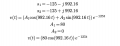#### MrAl

Joined Jun 17, 2014
10,586
Hello again,

How did you determine that A2 should be equal to zero?

#### MrAl

Joined Jun 17, 2014
10,586
Example from my textbook, I'm sure I'm not grasping the reasoning behind it then, can you give me an hint?

View attachment 179632
Hi,

I have no idea what you are doing there.
When we assume a solution like
Vc=(A1*sin(wt)+A2*cos(wt))*e^-at

then we use standard ways to find A1 and A2. I am not sure what you are doing.

However, one of the facts of this circuit is that at t=0 the first derivative of Vc(t) is zero and so when you find the solution you should see the cap voltage start out with a slope of zero and then gradually change as time progresses. Since A1 is not equal to zero using A2=0 i dont think you get that. You have to satisfy both the value of Vc(t) at t=0 and the first derivative of Vc(t) at t=0.

So you will either have to use a different method or explain your method in detail so we can see where you went wrong.

LATER:
Just to note, i went over this one more time and verified that your second order DE is correct. Your second coefficient however is not correct, but your first one (40) is correct. The coefficient of the cosine term in the time solution is 40 but the coefficient of the sine term is not zero.

Last edited:

#### gianx80

Joined Jan 23, 2011
14
Hi,
then we use standard ways to find A1 and A2
My book uses the values of v(0) and its derivative, for example, but doesn't explain why.

EDIT

Something like this? EXAMPLE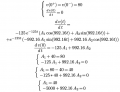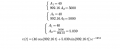Last edited:

#### MrAl

Joined Jun 17, 2014
10,586
My book uses the values of v(0) and its derivative, for example, but doesn't explain why.

EDIT

Something like this? EXAMPLE

View attachment 179971
View attachment 179975
Hi,

Yes that looks better now.
The procedure is like this...

1. Solve for one constant by setting t=0 in Vc(t). Use that constant in Vc(t)=Vc(0).
2. Using the new Vc(t), take the derivative and set t=0 again, then solve for the other constant with the derivative set to the known derivative at t=0.

So for one constant you use Vc(t) with t=0 and for the other you use dVc(t)/dt with t=0.

This brings up the question of how do we know what the derivative of Vc(t) is at t=0. We look at the circuit and realize that when the input changes, the current in the inductor can not change instantaneously therefore the capacitor voltage can not change in the first instant therefore the slope of Vc(t) near t=0 must be zero.
The first instant is the time between t=0 and t=0+ which is considered infinitesimal.
It may take a little time to becomes accustomed to this way of thinking for the initial values, but we just have to remember that with little time change 'dt' the current in an inductor can not change and the voltage across a capacitor can not change. That is key to understanding how to assume initial values.

Last edited:

#### gianx80

Joined Jan 23, 2011
14
Hi,

Yes that looks better now.
The procedure is like this...

1. Solve for one constant by setting t=0 in Vc(t). Use that constant in Vc(t)=Vc(0).
2. Using the new Vc(t), take the derivative and set t=0 again, then solve for the other constant with the derivative set to the known derivative at t=0.

So for one constant you use Vc(t) with t=0 and for the other you use dVc(t)/dt with t=0.

This brings up the question of how do we know what the derivative of Vc(t) is at t=0. We look at the circuit and realize that when the input changes, the current in the inductor can not change instantaneously therefore the capacitor voltage can not change in the first instant therefore the slope of Vc(t) near t=0 must be zero.
The first instant is the time between t=0 and t=0+ which is considered infinitesimal.
It may take a little time to becomes accustomed to this way of thinking for the initial values, but we just have to remember that with little time change 'dt' the current in an inductor can not change and the voltage across a capacitor can not change. That is key to understanding how to assume initial values.
So are you saying that my two equations for A1 and A2 are completely correct? Just to be sure ...

Thank you so much. You're explanation is very clear, I guess I understood the method. I hope I'll correctly apply it to other exercises. I'll write here again when I'm in needI'll try to calculate the current through the inductor (it was the real assignment) and I'll post here again tomorrow.

#### Plamen

Joined Mar 29, 2015
101
Hi to all and thanks in advance. I'm studying for one of my exams (I work so I can't attend lessons and the Professor doesn't reply to my emails).
In the following circuit I have to find the current through the inductor:

View attachment 179428

for t < 0 we have:

View attachment 179430

So IR1 = 10A, IR2 = 20A. Current through the inductor is 20A and the voltage across capacitor is 80V.

for t = ∞ we have:

View attachment 179429

So IR1 = 5A, IR2 = 10A. Current through the inductor is 10A and the voltage across capacitor is 40V.

for t = 0+ we have the initial circuit, the current though inductor is 20A and the voltage across the capacitor is 80V.
So we have a soluzione like this one:

View attachment 179431
now, the circuit is neither RLC parallel or series, right? Can I lead it back to a know simpler case? Am I doing something wrong?

I found examples with combination of series and parallels, but they use phasors and, in this case, I don't have a AC source (so I cannot compute impedance).

I found examples of RC or RL circuits solved used Thevenin, again, if I use the inductor as a load I have the parallel of a capacitor and a resistor, so no way.

How can I proceed?

Thanks, I'm desperate
Petkan:
If the E1 is an ideal voltage source, R1 is irrelevant. The steady state current is solely defined by the voltage source and the total resistance of the inductor and R2. However if the transient current is of interest, we have one initial steady state current (defined by V1=40V), and one final steady state current (defined by V1=80V), connected by exponentially rising curve. The exponent time constant L/R is defined by the inductance L and the total resistance of the inductor and R2. Here is a little simplification. Inductors act as current sources and dominate the current i.e. the output cap will have limited effect. Put it in LTSpice and see the difference